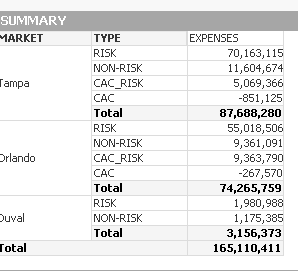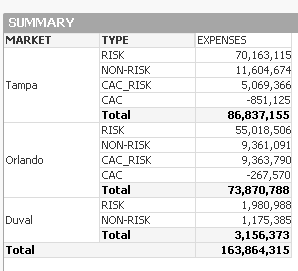# New to QlikView

Discussion board where members can get started with QlikView.

Announcements
Attend QlikWorld 2020 and hear keynote speaker, Malcolm Gladwell. Register by February 29th to save \$200. Learn More
Valued Contributor III

## In Pivot table, How to fabs first and then sum

Hi,     I’m trying to get the final result as sum of total for the +ve and –ve values. Please help.sunindia

Tags (1)
1 Solution

Accepted Solutions
HighlightedMVP

## Re: In Pivot table, How to fabs first and then sum

Maybe like

If(Dimensionality()<2,

Sum(Aggr(

If(SUM( [TOTAL EXPENSES])>0,SUM( [TOTAL EXPENSES]))

,MARKET,Risk_Type))

,

SUM([TOTAL EXPENSES]))

12 Replies
HighlightedMVP

## Re: In Pivot table, How to fabs first and then sum

If you make it positive, I get a little different number using this:

If(Dimensionality() = 2, SUM([TOTAL EXPENSES]), Sum(fabs([TOTAL EXPENSES])))HighlightedMVP

## Re: In Pivot table, How to fabs first and then sum

The answers matches your if we completely exclude negative from the calculation

If(Dimensionality() = 2, SUM([TOTAL EXPENSES]), Sum({<[TOTAL EXPENSES] = {'>0'}>}[TOTAL EXPENSES]))Highlighted
Valued Contributor III

## Re: Hi, I’m trying to get the final result as sum of total for the +ve and –ve values. Please help.

Hi Kumar

check the link -ve to +ve convertion

*** Negative Value to Positive Value ***

HighlightedMVP

## Re: In Pivot table, How to fabs first and then sum

If you are trying to show the sum-of-rows (with absolute values) for the partial sums, but still also negative values for the details, I would suggest that you create a field in your data model

...

if( left([VENDOR ID],3)= 'CPM' and Risk_Type = 'RISK', 'CAC_RISK', Risk_Type) as New_Risk_Type,

...

Then in your chart, use this new field as dimension and as expressions

=If(Dimensionality()<2,

Sum(Aggr(

Fabs(SUM( [TOTAL EXPENSES]))

,MARKET,New_Risk_Type))

,

SUM([TOTAL EXPENSES]))

Highlighted
Valued Contributor III

## Re: In Pivot table, How to fabs first and then sum

‌Bro.. Can you check the total for orlando. It should be 73,743,387 when we exclude the negative value completely...

Highlighted
Valued Contributor III

## Re: In Pivot table, How to fabs first and then sum

‌Bro.. Can you check the total for orlando. It should be 73,743,387 when we exclude the negative value completely... Please help...

Highlighted
Valued Contributor III

## Re: In Pivot table, How to fabs first and then sum

hi swehul,

s‌till I'm getting the wrong total.

HighlightedMVP

## Re: In Pivot table, How to fabs first and then sum

Could you upload the changed QVW with the new dimension and could you also detail what you expect to see, i.e. which lines should show which values and how you derive the values from your original table?

Highlighted
Valued Contributor III

## Re: In Pivot table, How to fabs first and then sum

‌As per my requirement, it should exclude the negative value from the total.